Ian finds patterns in the ratios and differences

of powers of powers, and gets an infinite sequence that approaches e.

A little background: From the 2nd grade when Ian first came to The Math Program, he was always looking for patterns, using differences and ratios. When Ian was here in about his 10th year in The Math Program, he worked on powers of powers. Don gave him a copy of the article "Polypowers" from Martin Gardner's book "Knotted Doughnuts and other Mathematical Entertainments" (published by W.H. Freeman and Company, NY, 1986). Subsequently, Ian was playing around with his calculator in his Physics class. Here is the result of this "playing around".

In line 1 below, when x = 2, he found (2+1)(2+1) = 33 = 27 and for line 2 when x = 3, he got (3+1)(3+1) = 44 = 256 and so on.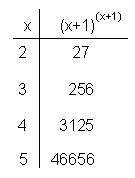He then found the differences of these powers.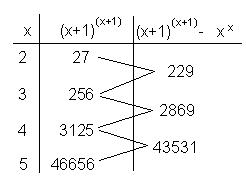Ian saw that the differences where getting very big, like the powers themselves. So he decided to look at the ratios of the powers. He noticed that the ratios do not get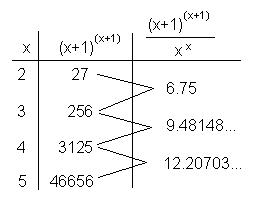very big and the differences of these ratios might lead to something.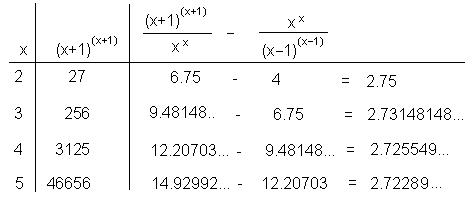He noticed that these differences on the right formed an infinite sequence approaching e. So his function is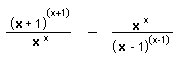and the limit as x goes to infinity or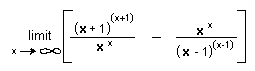= e   (WOW!)

Ian graphed his function in Mathematica,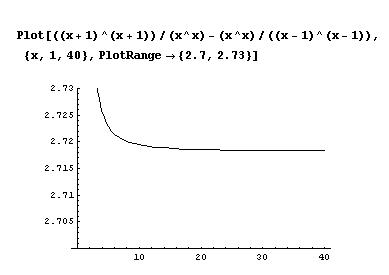an infinite sequence which approaches 2.71828... which = e

Great job Ian!!

Don had never seen this before, nor since.

Notice: e is also defined as the limitn->inf(1+1/n) [read this as "the limit of (1+1/n)n as n goes to infinity"] and can be written as an infinite series

To other discoveries

Back to Patterns in Numbers
To order Don's materials
Mathman home